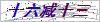# 温度传感器wzp-440

## 简要描述：免费咨询：0550-7307460发邮件给我们：1656350898@qq.com

## W温度传感器wzp-440.l=2米.-20--200.cWZP温度传感器选型 Pt100 铂热电阻 P口 0=Pt100     1= Pt1000     5=Pt500 铂热电阻类型 T口 0=0～80  1=-50～100  2=-50～150  3=-50～200    9=指定 温度范围(℃) 口 A=A级      B=B级 传感器精度 C口 1=SUS321(默认)   4=SUS304    6=SUS316    9=指定 保护管材质 L口 1=100   2=200   3=300   5=500  10=1000 30=3000    50=5000   9=指定 引线长度(mm) X口 2=两线制  3=三线制  4=四线制 引出线采用线制 口 1=聚氯乙烯PVC（-20～80℃） 2=聚氨酯TPU （-50～100℃）3=硅橡胶  （-60～200℃） 4=四氟绕包（-50～250℃）5=云母绕包 （-80～500℃）  9=指定 引线材质      “（）”内为引线常用温度范围 口 0=无屏蔽层（默认）    1=有屏蔽层 是否配屏蔽层 口 0=无护套          1=有护套 线缆护套 口 0=无         1=镀锡       3=压M4端子   9=指定 导线末端要求 Q 0=无    9=指定 特殊要求 说明：对于用户指定的参数要求，直接以数字或文字标注出来即可。选型举例：如选取类型为B级Pt100，温度0～50℃，保护管材质为SUS321导线为3000mm三线制无屏蔽层的聚氯乙烯线。

### 留言框

• #### 验证码：请输入计算结果（填写阿拉伯数字），如：三加四=7

QQ在线客服

18226997986

0550-7307460